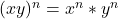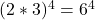## Using words and equations, explain what you learned about exponents in this lesson so that someone who was absent could read what you wrote

Question

Using words and equations, explain what you learned about exponents in this lesson so that someone who was absent could read what you wrote and understand the lesson. Consider using an example like 2^4 • 3^4 =6^4
I WILL MARK BRAINIEST FOR WHOEVER ANSWERS THE FASTEST

in progress 0
3 months 2021-08-17T16:17:13+00:00 1 Answers 1 views 0

Multiplication rule of exponents sayshence, 2^4 • 3^4 =6^4

Step-by-step explanation:

As per the rule of exponents,If we go by the above equation, we can deduce that x is 2 and y is 3

Thus, 2^4 *3^4 can be written asHence,  2^4 • 3^4 =6^4